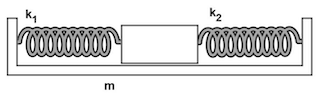# What will the frequency of the oscillation be when the mass is released?

## Question:The mass m = 11.3 kg shown in the figure is displaced a distance x = 0.149 m to the right from its equilibrium position. k₁ = 117 N/m and k₂ = 205 N/m.

What will the frequency of the oscillation be when the mass is released?

## Spring-Mass System:

Suppose a spring whose one end is rigidly fixed to the firm support, and to the other end, we connected a mass, and when we pull the mass away from its equilibrium position, then the spring employs restoring force on the mass. Once we release the mass, it starts oscillating, and the number of oscillations accomplished by the system in one second prominently depends on the stiffness of the system or effective stiffness as well as the mass attached to the spring.

Given data:

• {eq}m=\rm 11.3 \ kg {/eq} is the mass attached to the springs.
• {eq}k_1=\rm 117 \ N/m {/eq} is the spring constant (or stiffness) of the left spring.
• {eq}k_2=\rm 205 \ N/m {/eq} is the spring constant (or stiffness) of the right spring.
• {eq}x=\rm 0.149 \ m {/eq} is the deformation of each spring.

The amount of extension of the left spring and the amount of compression of the right spring is the same, so the given system act as springs in a parallel connection. The effective spring constant is:

{eq}\begin{align} k_{eq}&=k_1+k_2 \\[0.3cm] &=\rm 117 \ \frac{N}{m}+205 \ \frac{N}{m} \\[0.3cm] &=\rm 322 \ N/m \end{align} {/eq}

The frequency of oscillation of the mass is:

{eq}\begin{align} f&=\dfrac{1}{2\pi} \sqrt{\dfrac{k_{eq}}{m}} \\[0.3cm] &=\rm \dfrac{1}{2\pi} \sqrt{\dfrac{322 \ \frac{N}{m}}{11.3 \ kg}} \\[0.3cm] &\simeq \color{blue}{\boxed { \rm 0.850 \ Hz}} \ \ \ \ \ \rm (correct \ to \ three \ significant \ figures ) \end{align} {/eq}

Therefore, the frequency of the oscillation of the system is {eq}\color{blue}{\boxed { \rm 0.850 \ Hz}} {/eq}.Practice Applying Spring Constant Formulas

from

Chapter 17 / Lesson 11
3.4K

In this lesson, you'll have the chance to practice using the spring constant formula. The lesson includes four problems of medium difficulty involving a variety of real-life applications.